## Velocity Distribution of Fluid Between Rotating Pipes

Consider a fluid of viscosity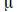between concentric rotating pipes of radius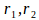with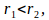rotating with angular velocities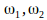respectively.

For two dimensional flow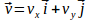in the z – direction the shear stress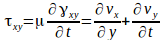where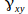is the shear strain and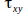is the shear stress.

If the flow is smooth and steady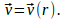For a particle at some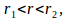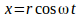and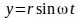where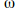is the angular velocity of the fluid at radius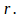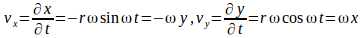.Hence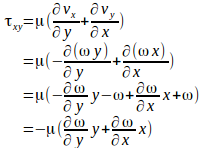If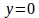then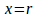and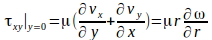Similarly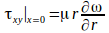Hence constant shear stress curves may be represented by concentric circles.

The torque acting on an element of fluid is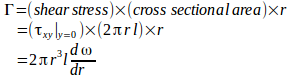This torque must be independent of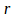since the flow is steady so there is no angular acceleration and the torque betweenand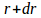must be zero. The torque above must be inependent ofso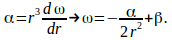At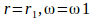and at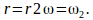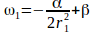(1) and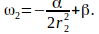subtraction gives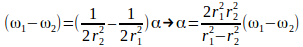Then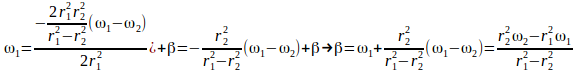Then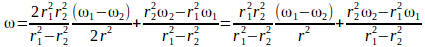Using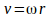gives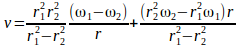.